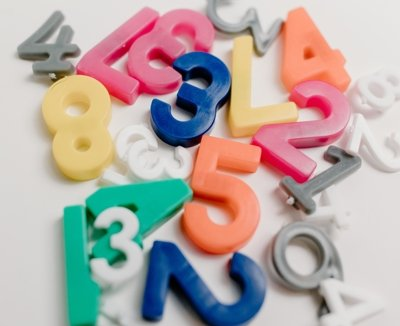Decimal to binary conversion is the process of converting a decimal number to its binary equivalent, where the binary number system has a base of 2 and only uses two digits (0 and 1). This conversion can be done by dividing the decimal number by 2 and keeping track of the remainder until the quotient becomes zero, and then arranging the remainders in reverse order to obtain the binary equivalent.

To convert a decimal number to binary:

1. Divide the decimal number by 2.
2. Write down the remainder (which will be either 0 or 1).
3. Continue dividing the quotient (the result of the division) by 2 and writing down the remainders until the quotient becomes zero.
4. Write the remainders in reverse order to obtain the binary equivalent.

For example, let’s convert the decimal number 27 to binary:

1. 27 divided by 2 gives a quotient of 13 with a remainder of 1. Write down the remainder: 1.
2. 13 divided by 2 gives a quotient of 6 with a remainder of 1. Write down the remainder: 1.
3. 6 divided by 2 gives a quotient of 3 with a remainder of 0. Write down the remainder: 0.
4. 3 divided by 2 gives a quotient of 1 with a remainder of 1. Write down the remainder: 1.
5. 1 divided by 2 gives a quotient of 0 with a remainder of 1. Write down the remainder: 1.

The remainders in reverse order are 1, 1, 0, 1, 1, which is the binary equivalent of 27: 11011.

This method can be used to convert any decimal number to binary. Note that the maximum number of bits required to represent a decimal number in binary is log2(n), where n is the decimal number.

Exemple in Javascript code:

``````function decimalToBinary(decimal) {
return (decimal >>> 0).toString(2);
}``````

Exemple in C# code:

``````public string DecimalToBinary(int decimalNumber)
{
if (decimalNumber == 0)
return "0";
else
{
string binary = "";
while (decimalNumber > 0)
{
int remainder = decimalNumber % 2;
binary = remainder.ToString() + binary;
decimalNumber /= 2;
}
return binary;
}
}``````

Categories:

Tags:

## Comentários

Nenhum comentário para mostrar.